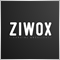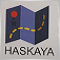# How to get Moving Average Cross price312

I am writing an EA that uses crossing moving averages. I tell the crossing of moving averages in the following way:

```// if shift5MA > shift0MA
if (shift5MAArray > shift0MAArray) {

//if shift5MA < shift0MA VALID SELL
if (shift5MAArray < shift0MAArray) {
signal = "sell";
}
}

if (shift5MAArray < shift0MAArray) {

//if shift5MA > shift0MA
if (shift5MAArray > shift0MAArray) {
}
}```

How can I tell the exact price at the point that moving averages cross, preferably after a candle closes?3125284

Jackery:

I am writing an EA that uses crossing moving averages. I tell the crossing of moving averages in the following way:

How can I tell the exact price at the point that moving averages cross, preferably after a candle closes?

```double   MA30  =  iMA(NULL,TMF,MAPeriod1,0,MODE_LWMA,PRICE_MEDIAN,0);
double   MA15  =  iMA(NULL,TMF,MAPeriod4,0,MODE_LWMA,PRICE_MEDIAN,0);

if (OrdersTotal()==0)
{
if ( (MA15 > MA30 && OldSignal !="BUY") )
{
}
if ( (MA15 < MA30 && OldSignal !="SELL") )
{
// sell here
OldSignal = "SELL";
}
}```

and define "OldSignal" variable on top off your code, global variable25339

1. Faeze Bakhshayesh:

That is not checking for a cross.

2. Jackery: How can I tell the exact price at the point that moving averages cross, preferably after a candle closes?

Search new bar. Stop checking each tick.

3. Exact price where they crossed? You can easily estimate that with algebra: f(t) = f + (f-f) × t, s(t) = s + (s-s) × t. At cross, they are equal and so is t.

4. Or the price when they crossed? Either use 1 and 0 and note the Bid on first cross. Or much harder binary search on price.

5. Why do you care? The market has moved away from either (№ 3 or 4) at candle close.5284

William Roeder:
1. That is not checking for a cross.

2. Search new bar. Stop checking each tick.

3. Exact price where they crossed? You can easily estimate that with algebra: f(t) = f + (f-f) × t, s(t) = s + (s-s) × t. At cross, they are equal and so is t.

4. Or the price when they crossed? Either use 1 and 0 and note the Bid on first cross. Or much harder binary search on price.

5. Why do you care? The market has moved away from either (№ 3 or 4) at candle close.

You can find crossing 2 ma with hundred of ways :)312

William Roeder:
1. That is not checking for a cross.

2. Search new bar. Stop checking each tick.

3. Exact price where they crossed? You can easily estimate that with algebra: f(t) = f + (f-f) × t, s(t) = s + (s-s) × t. At cross, they are equal and so is t.

4. Or the price when they crossed? Either use 1 and 0 and note the Bid on first cross. Or much harder binary search on price.

5. Why do you care? The market has moved away from either (№ 3 or 4) at candle close.

Sorry, I've not been here for a while.

I am trying to figure out how to use the equation you've provided in my code. I don't really get the understand what s(t) and f(t) represent with respect to the code I provided.

From my observation and backtesting, based on some other additional conditions, price might return to fill in orders at this cross price before continuing in it's respective direction.312

Faeze Bakhshayesh:

You can find crossing 2 ma with hundred of ways :)

I would really appreciate you showing me one of those hundred ways :)312

I would really appreciate some help with getting this solved2682

Faeze Bakhshayesh:

and define "OldSignal" variable on top off your code, global variable

```double   MA301  =  iMA(NULL,TMF,MAPeriod1,0,MODE_LWMA,PRICE_MEDIAN,0);
double   MA302  =  iMA(NULL,TMF,MAPeriod1,0,MODE_LWMA,PRICE_MEDIAN,1);

double   MA151  =  iMA(NULL,TMF,MAPeriod4,0,MODE_LWMA,PRICE_MEDIAN,0);
double   MA152  =  iMA(NULL,TMF,MAPeriod4,0,MODE_LWMA,PRICE_MEDIAN,1);

if (OrdersTotal()==0)
{
if ( (MA151 > MA301 && MA152 < MA302 && OldSignal !="BUY") )
{